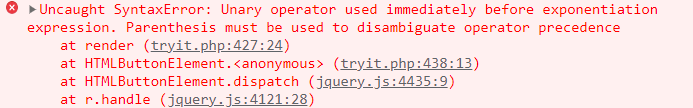GFG App
Open AppBrowser
Continue

# Exponentiation(**) Arithmetic Operator in JavaScript

JavaScript exponentiation(**) operator in JavaScript is represented by “**” and is used to find the power of the first operator raised to the second operator. This operator is equal to Math.pow() but makes the code simpler and can even accept BigInt primitive data type. The exponentiation operator is right associative which means that x**y**z will give the same result as x**(y**z).

Syntax:

`a**b`

Return: It returns the result of raising the first operand to the power of the second operand.

Example 1: In this example, we will use the exponentiation operator on positive numbers.

## Javascript

 `let a=4; ` `let b=3; ` `let c=-2 ` `console.log(a**b); ` `console.log(b**a); ` `console.log(c**a); ` `console.log(a**c)`

Output:

```64
81
16
0.0625```

Example 2: In this example, we will try to find the powers of a negative number before assigning it to a variable

## Javascript

 `console.log(-2**3)`

Output:Explanation: Like other languages, JavaScript does not allow a unary operator before exponentiation expression to run this program. To overcome this problem we will have to put -2 in parenthesis like (-2)

Example 3: In this example, we will try to find the power of NaN

## Javascript

 `console.log(NaN**3) ` `console.log(NaN**0)`

Output:

```NaN
1```

Note: In some languages, ‘^’ operator is used as an exponentiation operator but JavaScript uses this operator as bitwise logical XOR operator.

Supported Browser:

• Chrome
• Edge
• Firefox
• Opera
• Safari

My Personal Notes arrow_drop_up